## 2.5 5NG#3: Histograms

Let’s consider the temp variable in the weather data frame once again, but unlike with the linegraphs in Section 2.4, let’s say we don’t care about its relationship with time, but rather we only care about how the values of temp distribute. In other words:

1. What are the smallest and largest values?
2. What is the “center” or “most typical” value?
3. How do the values spread out?
4. What are frequent and infrequent values?

One way to visualize this distribution of this single variable temp is to plot them on a horizontal line as we do in Figure 2.8: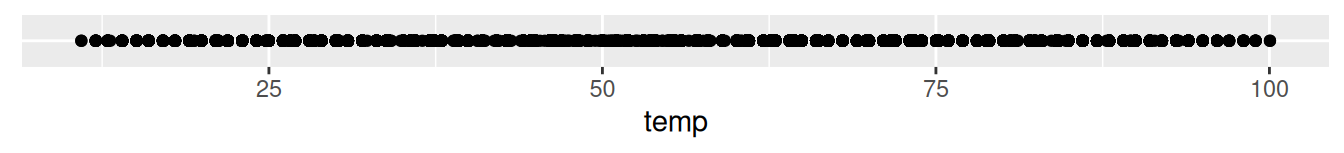FIGURE 2.8: Plot of hourly temperature recordings from NYC in 2013.

This gives us a general idea of how the values of temp distribute: observe that temperatures vary from around 11°F (-11°C) up to 100°F (38°C). Furthermore, there appear to be more recorded temperatures between 40°F and 60°F than outside this range. However, because of the high degree of overplotting in the points, it’s hard to get a sense of exactly how many values are between say 50°F and 55°F.

What is commonly produced instead of Figure 2.8 is known as a histogram. A histogram is a plot that visualizes the distribution of a numerical value as follows:

1. We first cut up the x-axis into a series of bins, where each bin represents a range of values.
2. For each bin, we count the number of observations that fall in the range corresponding to that bin.
3. Then for each bin, we draw a bar whose height marks the corresponding count.

Let’s drill-down on an example of a histogram, shown in Figure 2.9.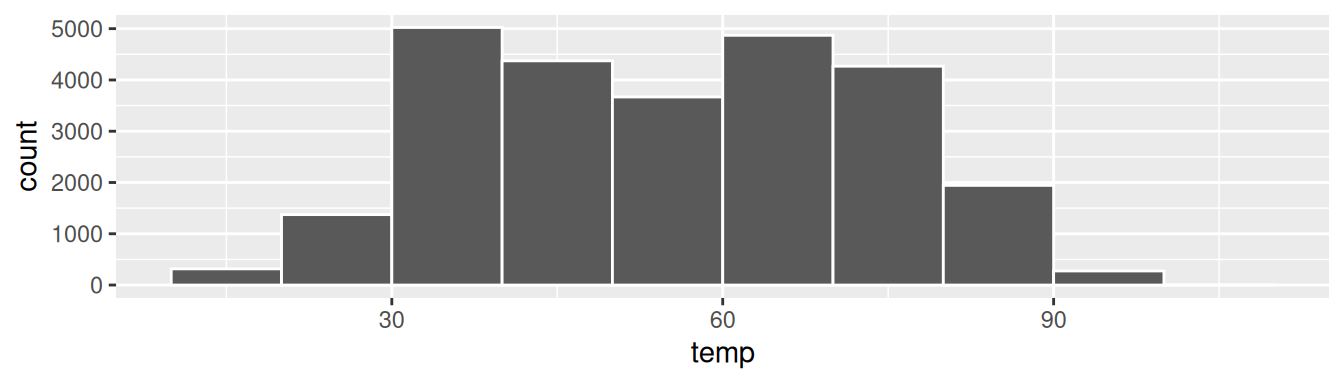FIGURE 2.9: Example histogram.

Let’s focus only on temperatures between 30°F (-1°C) and 60°F (15°C) for now. Observe that there are three bins of equal width between 30°F and 60°F. Thus we have three bins of width 10°F each: one bin for the 30-40°F range, another bin for the 40-50°F range, and another bin for the 50-60°F range. Since:

1. The bin for the 30-40°F range has a height of around 5000. In other words, around 5000 of the hourly temperature recordings are between 30°F and 40°F.
2. The bin for the 40-50°F range has a height of around 4300. In other words, around 4300 of the hourly temperature recordings are between 40°F and 50°F.
3. The bin for the 50-60°F range has a height of around 3500. In other words, around 3500 of the hourly temperature recordings are between 50°F and 60°F.

All nine bins spanning 10°F to 100°F on the x-axis have this interpretation.

### 2.5.1 Histograms via geom_histogram

Let’s now present the ggplot() code to plot your first histogram! Unlike with scatterplots and linegraphs, there is now only one variable being mapped in aes(): the single numerical variable temp. The y-aesthetic of a histogram, the count of the observations in each bin, gets computed for you automatically. Furthermore, the geometric object layer is now a geom_histogram(). After running the following code, you’ll see the histogram in Figure 2.10 as well as warning messages. We’ll discuss the warning messages first.

ggplot(data = weather, mapping = aes(x = temp)) +
geom_histogram()
stat_bin() using bins = 30. Pick better value with binwidth.
Warning: Removed 1 rows containing non-finite values (stat_bin).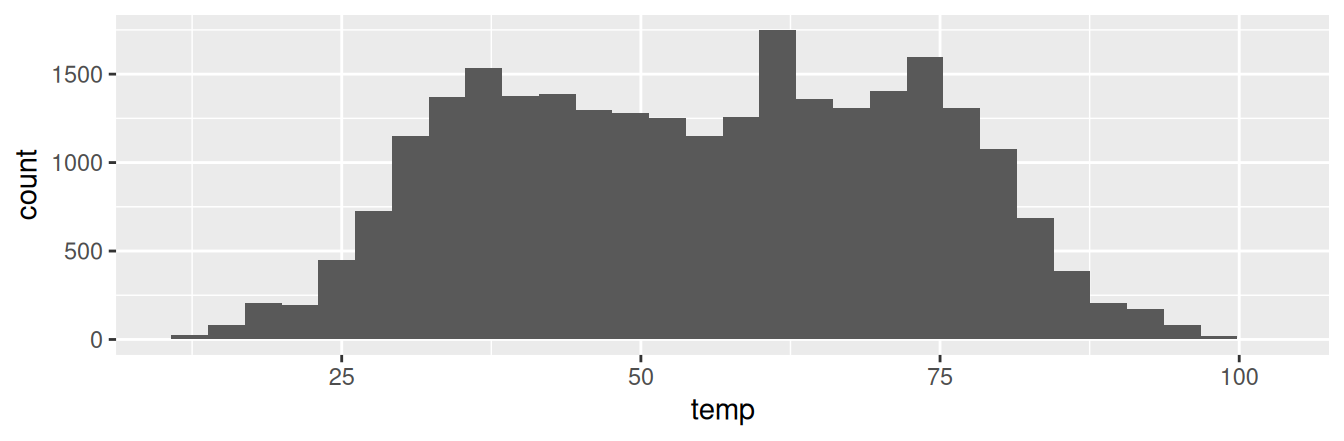FIGURE 2.10: Histogram of hourly temperatures at three NYC airports.

The first message is telling us that the histogram was constructed using bins = 30 for 30 equally spaced bins. This is known in computer programming as a default value; unless you override this default number of bins with a number you specify, R will choose 30 by default. We’ll see in the next section how to change the number of bins to another value than the default.

The second message is telling us something similar to the warning message we received when we ran the code to create a scatterplot of departure and arrival delays for Alaska Airlines flights in Figure 2.2: that because one row has a missing NA value for temp, it was omitted from the histogram. R is just giving us a friendly heads up that this was the case.

Now let’s unpack the resulting histogram in Figure 2.10. Observe that values less than 25°F as well as values above 80°F are rather rare. However, because of the large number of bins, it’s hard to get a sense for which range of temperatures is spanned by each bin; everything is one giant amorphous blob. So let’s add white vertical borders demarcating the bins by adding a color = "white" argument to geom_histogram() and ignore the warning about setting the number of bins to a better value:

ggplot(data = weather, mapping = aes(x = temp)) +
geom_histogram(color = "white")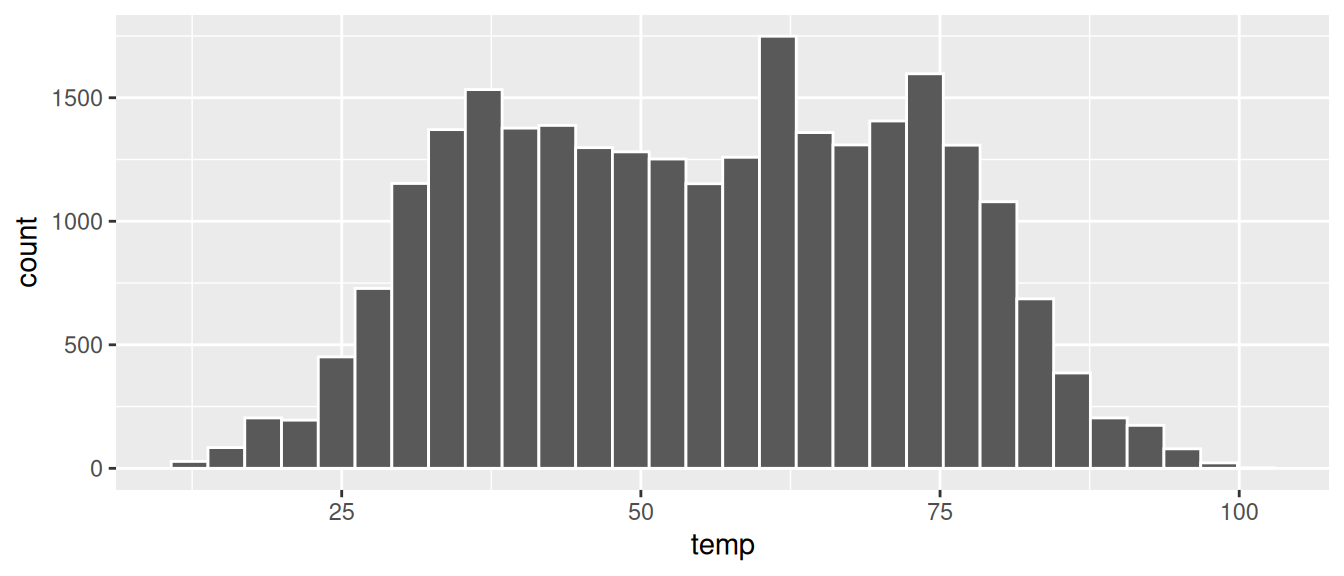FIGURE 2.11: Histogram of hourly temperatures at three NYC airports with white borders.

We now have an easier time associating ranges of temperatures to each of the bins in Figure 2.11. We can also vary the color of the bars by setting the fill argument. For example, you can set the bin colors to be “blue steel” by setting fill = "steelblue":

ggplot(data = weather, mapping = aes(x = temp)) +
geom_histogram(color = "white", fill = "steelblue")

If you’re curious, run colors() to see all 657 possible choice of colors in R!

Observe in Figure 2.11 that in the 50-75°F range there appear to be roughly 8 bins. Thus each bin has width 25 divided by 8, or 3.125°F, which is not a very easily interpretable range to work with. Let’s improve this by adjusting the number of bins in our histogram in one of two ways:

1. By adjusting the number of bins via the bins argument to geom_histogram().
2. By adjusting the width of the bins via the binwidth argument to geom_histogram().

Using the first method, we have the power to specify how many bins we would like to cut the x-axis up in. As mentioned in the previous section, the default number of bins is 30. We can override this default, to say 40 bins, as follows:

ggplot(data = weather, mapping = aes(x = temp)) +
geom_histogram(bins = 40, color = "white")

Using the second method, instead of specifying the number of bins, we specify the width of the bins by using the binwidth argument in the geom_histogram() layer. For example, let’s set the width of each bin to be 10°F.

ggplot(data = weather, mapping = aes(x = temp)) +
geom_histogram(binwidth = 10, color = "white")

We compare both resulting histograms side-by-side in Figure 2.12.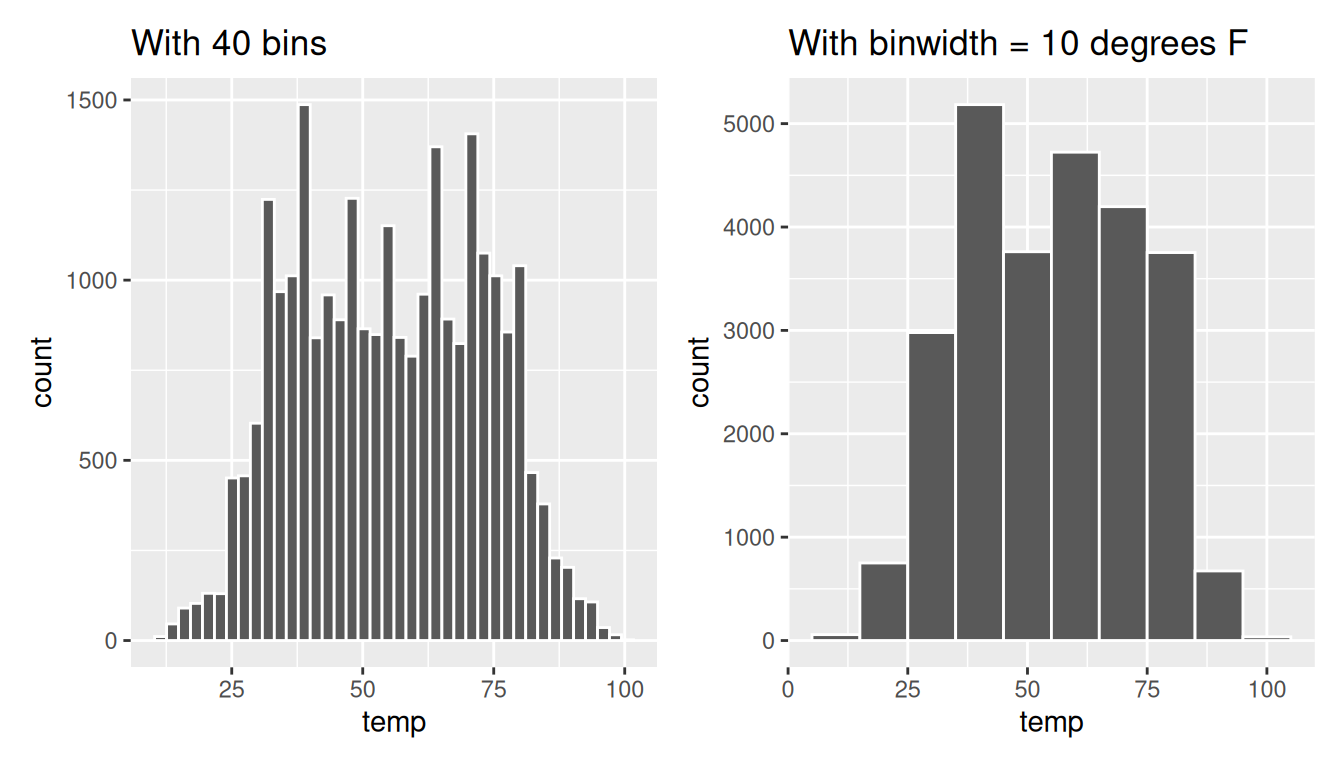FIGURE 2.12: Setting histogram bins in two ways.

Learning check

(LC2.14) What does changing the number of bins from 30 to 40 tell us about the distribution of temperatures?

(LC2.15) Would you classify the distribution of temperatures as symmetric or skewed in one direction or another?

(LC2.16) What would you guess is the “center” value in this distribution? Why did you make that choice?

(LC2.17) Is this data spread out greatly from the center or is it close? Why?

### 2.5.3 Summary

Histograms, unlike scatterplots and linegraphs, present information on only a single numerical variable. Specifically, they are visualizations of the distribution of the numerical variable in question.